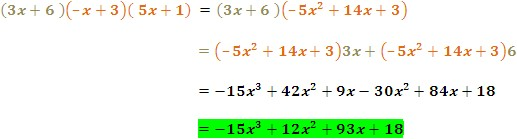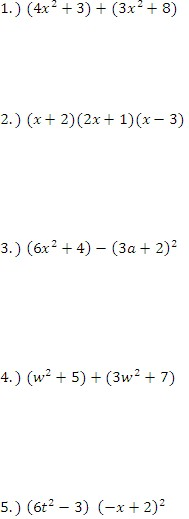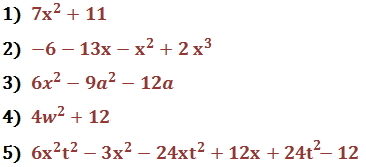To add or subtract polynomials, line up the coefficients with like terms and then add or subtract them.

Example

Add and subtract the following two polynomials.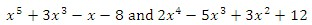a.) To add, line up like terms and then add the polynomials.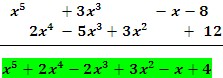b.) To subtract, line up like terms, distribute the negative sign, and add them together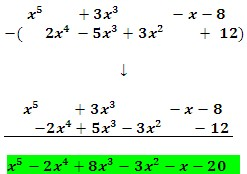Multiply Polynomials

To multiply two polynomials, each term of the first polynomial must be multiplied by each term of the second polynomial.

Example

Multiply the following polynomials: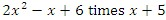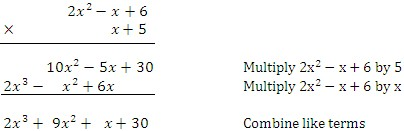You can also multiply terms horizontally.

Example

Multiply the following polynomials: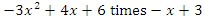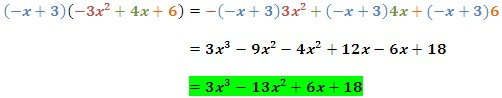When multiplying binomial products, there are some special product patterns that are worth keeping in mind. These are the special product patterns: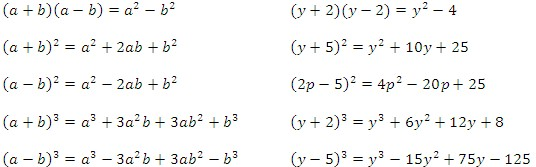You can also multiply more than two binomials.

Example

Multiply the following polynomials:

3x + 6 times - x + 3 times 5x + 1